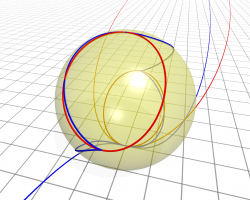# Number TheoryNumber theory is the study of integers and fractions (rational numbers) – how they factor and how they arise as solutions to equations.

### Our areas of expertise

Diophantine analysis, number fields, polynomial equations in several variables, algebraic number theory and p-adic analysis, elliptic curves and Iwasawa theory. Interdisciplinary interests include cryptography and information security.